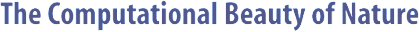Computer Explorations of Fractals, Chaos,Complex Systems, and Adaptation· title page · home* · cover artwork · jacket text · table of contents · the author* · ordering informationBook Contents
 · three themes · part synopses · selected excerpts · all figures from book · quotes from book · glossary from book · bibliography · slide showSource Code
 · overview &documentation · FAQ list* · download source code · java appletsMiscellany
 · news* · reviews & awards · errata · for educators · bibliography (BibTeX format) · other linksGAIPD Documentation

```

```

#### NAME

```       gaipd - breed IPD strategies with a genetic algorithm

```

#### SYNOPSIS

```       gaipd -help
or
gaipd  [-size  integer]  [-gens  integer] [-bouts integer]
[-rounds integer] [-hlen integer]  [-seed  integer]
[-crate  double]  [-mrate  double]  [-noise double]
[-CC double] [-CD double] [-DC double] [-DD double]
[-dump]

```

#### DESCRIPTION

```       Use a genetic algorithm to evolve IPD strategies according
to user-specified constraints.  This  program  illustrates
how  GAs can demonstrate co-evolution since IPD strategies
can only be successful within the context of their  likely
opponents.   Reproduction  of strategies entails crossover
and mutation with strategies being selected based on  fit-
ness.

```

#### OPTIONS

```       -size integer
Population size.

-gens integer
Number of generations.

-bouts integer
Bouts per generation.

-rounds integer
Rounds per bout.

-hlen integer
History length.

-seed integer
Random seed.

-crate double
Crossover rate.

-mrate double
Mutation rate.

-noise double
Chance of mistake in transaction.

-CC double
Reward Payoff.

-CD double
Sucker Payoff.

-DC double
Temptation Payoff.

-DD double
Punish Payoff.

-dump  Print entire population at end?

```

#### PAYOFFS

```       The  payoff matrix for the Prisoner's Dilemma game is usu-
ally expressed as:
Player B's Move
+-----------+-----------+
Player A's Move | cooperate |  defect   |
+-----------+-----------+-----------+
| cooperate |  CC, CC   |  CD, DC   |
+-----------+-----------+-----------+
|    defect |  DC, CD   |  DD, DD   |
+-----------+-----------+-----------+

where the table entries are (A's payoff, B's  payoff)  and
CC, CD, DC, and DD are the reward, sucker, temptation, and
punish payoffs, respectively.  For each of these four out-
comes  you  will  probably want the payoffs to reflect the
relationships:

(DC > CC > DD > CD) and ((CD + DC) / 2 < CC).

```

#### GENERATIONS

```       A single generation proceeds as follows.  Each  member  of
the  population  must  play  several  bouts  with randomly
selected opponents.  For each opponent, several rounds are
played.  The total score after these bouts is a strategy's
raw fitness score.

```

#### STRINGS

```       Since population strings may be  optionally  displayed  at
the end of the simulation, this section describes the for-
mat of these strings.  Given two players, A and B, and the
current  time,  t, and letting cooperation be denoted by 0
and defection by 1, form a bit string such as:

A(t-1) B(t-1) A(t-2) B(t-2) ... A(t-H) B(t-H)

where A(T) and B(T) are A  and  B's  moves  from  time  T,
respectively,  and  H  is the number of time steps "remem-
bered" by each player.  This bit string can take 2^(2 * H)
values.  To define a complete strategy, we must have a H +
1 separate tables of this form to describe  each  possible
history.   Thus,  the rule table string used internally in
the program and displayed at the end have as  their  first
entry  the move to make with no previous history, followed
by four entries for H equals to  1,  followed  by  sixteen
entries for when H equals 2, and so on.

As an annotated example, Tit-for-Tat is encoded as "CCDCD"
so that the first "C" indicates that the first move should
be  C  while the last for characters indicate what to play
if A(t-1) and B(t-1) is equal to (C, C), (C, D),  (D,  C),
and (D, D), respectively.

```

#### HINTS

```       Without  any  parameters  (and assuming you have an uncor-
rupted version if this source code) running  this  program
without  any  parameters  will probably result in Tit-for-
Tat, "CCDCD", dominating. If you run it  with  the  -noise
0.1 option, then Pavlov, "CCDDC", will probably win in the
end since it is more resistant to noise.

See  the  author's  book,  "The  Computational  Beauty  of
Nature," for more details.

```

#### MISCELLANY

```       The  fitness  function  relies on two steps done after the
raw fitness scores are calculated.  The raw fitness  score
from  the  previous step is divided by the total number of
PD rounds played.  (Note that this may vary among  popula-
tion  members  since  opponents  are  selected at random.)
This yields a scaled fitness score.  The  normalized  fit-
ness  is then set to the scaled fitness divided by the sum
of the scaled fitnesses.  Thus the sum of  the  normalized
fitnesses must be equal to one.

```

#### BUGS

```       No  sanity  checks  are performed to make sure that any of
the options make sense.

```

#### AUTHOR

```       Copyright (c) 1997, Gary William Flake.

Permission granted for any use according to  the  standard
GNU ``copyleft'' agreement provided that the author's com-
ments are neither modified nor removed.   No  warranty  is
given or implied.

```Copyright © Gary William Flake, 1998-2002. All Rights Reserved.Last modified: 30 Nov 2002